Chemistry 2e

# 7.4Formal Charges and Resonance

Chemistry 2e7.4 Formal Charges and Resonance

### Learning Objectives

By the end of this section, you will be able to:

• Compute formal charges for atoms in any Lewis structure
• Use formal charges to identify the most reasonable Lewis structure for a given molecule
• Explain the concept of resonance and draw Lewis structures representing resonance forms for a given molecule

In the previous section, we discussed how to write Lewis structures for molecules and polyatomic ions. As we have seen, however, in some cases, there is seemingly more than one valid structure for a molecule. We can use the concept of formal charges to help us predict the most appropriate Lewis structure when more than one is reasonable.

### Calculating Formal Charge

The formal charge of an atom in a molecule is the hypothetical charge the atom would have if we could redistribute the electrons in the bonds evenly between the atoms. Another way of saying this is that formal charge results when we take the number of valence electrons of a neutral atom, subtract the nonbonding electrons, and then subtract the number of bonds connected to that atom in the Lewis structure.

Thus, we calculate formal charge as follows:

$formal charge=# valence shell electrons (free atom)−# lone pair electrons−12# bonding electronsformal charge=# valence shell electrons (free atom)−# lone pair electrons−12# bonding electrons$

We can double-check formal charge calculations by determining the sum of the formal charges for the whole structure. The sum of the formal charges of all atoms in a molecule must be zero; the sum of the formal charges in an ion should equal the charge of the ion.

We must remember that the formal charge calculated for an atom is not the actual charge of the atom in the molecule. Formal charge is only a useful bookkeeping procedure; it does not indicate the presence of actual charges.

### Example 7.6

#### Calculating Formal Charge from Lewis Structures

Assign formal charges to each atom in the interhalogen ion $ICl4−.ICl4−.$

#### Solution

1. Step 1. We divide the bonding electron pairs equally for all I–Cl bonds: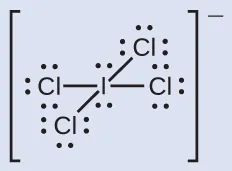2. Step 2. We assign lone pairs of electrons to their atoms. Each Cl atom now has seven electrons assigned to it, and the I atom has eight.
3. Step 3. Subtract this number from the number of valence electrons for the neutral atom:
I: 7 – 8 = –1
Cl: 7 – 7 = 0
The sum of the formal charges of all the atoms equals –1, which is identical to the charge of the ion (–1).

Calculate the formal charge for each atom in the carbon monoxide molecule: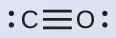C −1, O +1

### Example 7.7

#### Calculating Formal Charge from Lewis Structures

Assign formal charges to each atom in the interhalogen molecule BrCl3.

#### Solution

1. Step 1. Assign one of the electrons in each Br–Cl bond to the Br atom and one to the Cl atom in that bond: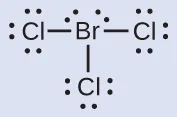2. Step 2. Assign the lone pairs to their atom. Now each Cl atom has seven electrons and the Br atom has seven electrons.
3. Step 3. Subtract this number from the number of valence electrons for the neutral atom. This gives the formal charge:
Br: 7 – 7 = 0
Cl: 7 – 7 = 0
All atoms in BrCl3 have a formal charge of zero, and the sum of the formal charges totals zero, as it must in a neutral molecule.

Determine the formal charge for each atom in NCl3.

N: 0; all three Cl atoms: 0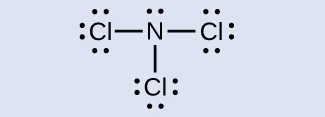### Using Formal Charge to Predict Molecular Structure

The arrangement of atoms in a molecule or ion is called its molecular structure. In many cases, following the steps for writing Lewis structures may lead to more than one possible molecular structure—different multiple bond and lone-pair electron placements or different arrangements of atoms, for instance. A few guidelines involving formal charge can be helpful in deciding which of the possible structures is most likely for a particular molecule or ion:

1. A molecular structure in which all formal charges are zero is preferable to one in which some formal charges are not zero.
2. If the Lewis structure must have nonzero formal charges, the arrangement with the smallest nonzero formal charges is preferable.
3. Lewis structures are preferable when adjacent formal charges are zero or of the opposite sign.
4. When we must choose among several Lewis structures with similar distributions of formal charges, the structure with the negative formal charges on the more electronegative atoms is preferable.

To see how these guidelines apply, let us consider some possible structures for carbon dioxide, CO2. We know from our previous discussion that the less electronegative atom typically occupies the central position, but formal charges allow us to understand why this occurs. We can draw three possibilities for the structure: carbon in the center and double bonds, carbon in the center with a single and triple bond, and oxygen in the center with double bonds: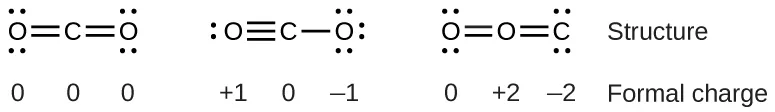Comparing the three formal charges, we can definitively identify the structure on the left as preferable because it has only formal charges of zero (Guideline 1).

As another example, the thiocyanate ion, an ion formed from a carbon atom, a nitrogen atom, and a sulfur atom, could have three different molecular structures: NCS, CNS, or CSN. The formal charges present in each of these molecular structures can help us pick the most likely arrangement of atoms. Possible Lewis structures and the formal charges for each of the three possible structures for the thiocyanate ion are shown here: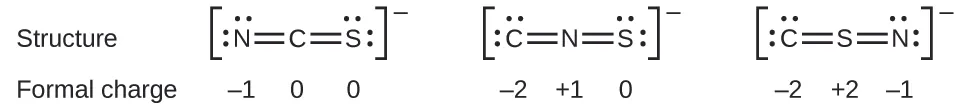Note that the sum of the formal charges in each case is equal to the charge of the ion (–1). However, the first arrangement of atoms is preferred because it has the lowest number of atoms with nonzero formal charges (Guideline 2). Also, it places the least electronegative atom in the center, and the negative charge on the more electronegative element (Guideline 4).

### Example 7.8

#### Using Formal Charge to Determine Molecular Structure

Nitrous oxide, N2O, commonly known as laughing gas, is used as an anesthetic in minor surgeries, such as the routine extraction of wisdom teeth. Which is the more likely structure for nitrous oxide?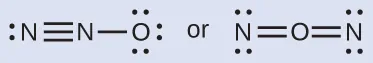#### Solution

Determining formal charge yields the following: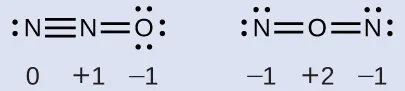The structure with a terminal oxygen atom best satisfies the criteria for the most stable distribution of formal charge: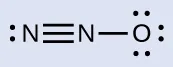The number of atoms with formal charges are minimized (Guideline 2), there is no formal charge with a magnitude greater than one (Guideline 2), the negative formal charge is on the more electronegative element (Guideline 4), and the less electronegative atom is in the center position.

Which is the most likely molecular structure for the nitrite $(NO2−)(NO2−)$ ion?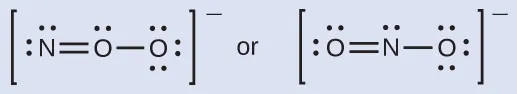ONO

### Resonance

Notice that the more likely structure for the nitrite anion in Example 7.8 may actually be drawn in two different ways, distinguished by the locations of the N-O and N=O bonds: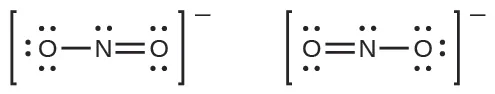If nitrite ions do indeed contain a single and a double bond, we would expect for the two bond lengths to be different. A double bond between two atoms is shorter (and stronger) than a single bond between the same two atoms. Experiments show, however, that both N–O bonds in $NO2−NO2−$ have the same strength and length, and are identical in all other properties.

It is not possible to write a single Lewis structure for $NO2−NO2−$ in which nitrogen has an octet and both bonds are equivalent. Instead, we use the concept of resonance: if two or more Lewis structures with the same arrangement of atoms can be written for a molecule or ion, the actual distribution of electrons is an average of that shown by the various Lewis structures. The actual distribution of electrons in each of the nitrogen-oxygen bonds in $NO2−NO2−$ is the average of a double bond and a single bond. We call the individual Lewis structures resonance forms. The actual electronic structure of the molecule (the average of the resonance forms) is called a resonance hybrid of the individual resonance forms. A double-headed arrow between Lewis structures indicates that they are resonance forms.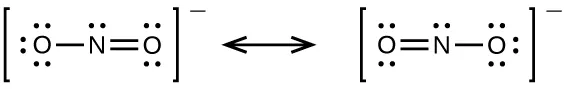We should remember that a molecule described as a resonance hybrid never possesses an electronic structure described by either resonance form. It does not fluctuate between resonance forms; rather, the actual electronic structure is always the average of that shown by all resonance forms. George Wheland, one of the pioneers of resonance theory, used a historical analogy to describe the relationship between resonance forms and resonance hybrids. A medieval traveler, having never before seen a rhinoceros, described it as a hybrid of a dragon and a unicorn because it had many properties in common with both. Just as a rhinoceros is neither a dragon sometimes nor a unicorn at other times, a resonance hybrid is neither of its resonance forms at any given time. Like a rhinoceros, it is a real entity that experimental evidence has shown to exist. It has some characteristics in common with its resonance forms, but the resonance forms themselves are convenient, imaginary images (like the unicorn and the dragon).

The carbonate anion, $CO32−,CO32−,$ provides a second example of resonance:One oxygen atom must have a double bond to carbon to complete the octet on the central atom. All oxygen atoms, however, are equivalent, and the double bond could form from any one of the three atoms. This gives rise to three resonance forms of the carbonate ion. Because we can write three identical resonance structures, we know that the actual arrangement of electrons in the carbonate ion is the average of the three structures. Again, experiments show that all three C–O bonds are exactly the same.

Order a print copy

As an Amazon Associate we earn from qualifying purchases.